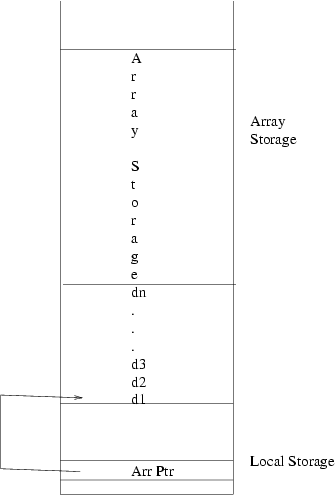# CPSC 411 - Lab Notes - 04-14 (Array Generation)

## How an array looks on the stack.## Array Generation.

The gencode method for iArray must create elements on the stack to match the above. Recall that it has two pieces of information we need, the offset and expression_list. (It currently also holds the array type. This is not needed in this language as each data type takes the same amount of storage, 1 cell.)

From this, we need to compute each of the dimensions, place them on top of the stack, allocate enough space for the array data and update the deallocation counter.

First, we place the first dimension on top of the stack and store a pointer to it in the array variable.

```self.expression_list.codegen(function_level)
print "STORE_O %d"%self.offset
```

Next, we loop through the rest of the dimensions, placing them on the stack.

```for dim in self.expression_list[1:]:
dim.codegen(function_level)
```

Note that due to the potential of side-effects, we may only compute the dimensions once. Since we need to save them as part of the array and we need to compute the total storage with them, we need to duplicate them on top of the stack.

```number_of_dims = length(self.expression_list)
for i in range(number_of_dims):
print "LOAD_O -%d"%(number_of_dims -1) #const offset from tos.
```

We can now multiply these new copies together.

```print (number_of_dims - 1)*"APP MUL\n",
```

Now we have the total amount we want to allocate on the top of the stack. But before we allocate, we must update the deallocation counter. We adjust the dealloc counter by this product + the number of dimensions. First, we must duplicate it as we need to save the value for later. Then, we add the number of dimensions.

```print "LOAD_R %sp"
```

Now, we bring the dealloc counter to the top of the stack, subtract (as we always store a negative value in the dealloc counter) and replace it in the stack.

```print "LOAD_R %fp"
print "APP SUB"
print "STORE_O 0"
```

This leaves the size on top of the stack, we now allocate.

```print "ALLOC_S"
```

## Array element access

This algorithm is used whenever you access an array element, whether in a read, assign or expression. (Note this is slightly expanded from what we did in class.) This is to calculate the array address of a reference. For example if we had `var x;`, then the formula for accessing `x[i][j][k]` is `3+i*12*3+j*3+k` which can be calculated as we loop through the addresses.

1. Load 0 on top of the stack.
2. Loop through the array address list: (Hint - do this in reverse order)
• Generate the code for the array address expression.
Last modified: Wed Apr 16 08:58:29 MDT 2003